batgrbproduct analysis for trigger=781740

## batgrbproduct Analysis for Trigger=781740

### Notes:

• 1) No attempt has been made to eliminate the non-significant decimal places.
• 2) This batgrbproduct analysis is done of the amount of data for this trigger downloaded to date.
• 3) This batgrbproduct analysis is using the flight position for the mask-weighting calculation.
```======================================================================
BAT GRB EVENT DATA PROCESSING REPORT
Process Script Ver: batgrbproduct v2.46
Process Start Time (local time): Tue Oct 24 11:12:41 EDT 2017
Host Name: bat1
======================================================================

Trigger: 00781740      Segment: 000

BAT Trigger Time: 530440458.56   [s; MET]
Trigger Stop: 530440459.584   [s; MET]
UTC: 2017-10-23T08:33:58.675420    [includes UTCF correction]

BAT
RA: 10.6330848310285   Dec: 41.1650551179965  [deg; J2000]

Catalogged Source?: YES
Point Source?:      YES
GRB Indicated?:     NO    [ by BAT flight software ]
Image S/N Ratio:    5.93
Image Trigger?:     NO
Rate S/N Ratio:     26.5706605111728  [ if not an image trigger ]
Image S/N Ratio:    5.93

Flight Position:   [ source = BAT ]
RA: 10.6330848310285   Dec: 41.1650551179965  [deg; J2000]

Ground Position:   [ source = BAT pre-slew burst ]
RA: 10.6593153962933   Dec: 41.1434831765995  [deg; J2000]
{ 00h 42m 38.2s , +41d 08' 36.5" }
+/- 3.0739140686614 [arcmin] (estimated 90% radius based on SNR)
+/- 1.42961372425083 [arcmin] (formal 1-sigma fit error)
SNR: 6.11527397692301

Angular difference between Flight and Ground position is 1.754847 arcmin

Partial Coding Fraction: 0.6328125    [ including projection effects ]

Duration
T90: 0.915999948978424 +/- 0.260376657598808
Measured from: 0.0160000920295715
to: 0.932000041007996     [s; relative to TRIGTIME]
T50: 0.539999961853027 +/- 0.277243547135739
Measured from: 0.232000112533569
to: 0.772000074386597     [s; relative to TRIGTIME]

Fluence
Peak Flux  (peak 1 second)
Measured from: -0.0300000309944153
to: 0.969999969005585     [s; relative to TRIGTIME]
Total Fluence
Measured from: -0.0239999890327454
to: 0.995999991893768     [s; relative to TRIGTIME]

Band 1     Band 2     Band 3     Band 4
15-25      25-50     50-100    100-350     keV
Total     0.034136   0.053683   0.004685   0.010349
0.009490   0.009635   0.006731   0.005445 [error]
Peak      0.031647   0.050196   0.005794   0.011071
0.009380   0.009517   0.006674   0.005393 [error]

[ fluence units of on-axis counts / fully illuminated detector ]

======================================================================

=== Fluence/Peak Flux Summary ===

Model    Band1    Band2    Band3    Band4    Band5    Total
15-25    25-50   50-100  100-350   15-150   15-350   keV
Fluence   Power-law 1.16e-08 1.41e-08 1.23e-08 1.84e-08 4.44e-08 5.64e-08   erg/cm2
Error 3.90e-09 4.00e-09 5.78e-09 1.65e-08 1.44e-08 2.46e-08   erg/cm2
Peak flux Power-law 3.51e-01 2.46e-01 1.13e-01 7.30e-02 7.45e-01 7.83e-01   ph/cm2/s
Error 1.28e-01 7.03e-02 5.18e-02 6.17e-02 2.11e-01 2.23e-01   ph/cm2/s

Fluence   Cutoff-PL 9.34e-09 2.25e-08 5.72e-09 3.80e-11 3.76e-08 3.76e-08   erg/cm2
Error 7.10e-09 7.04e-09 6.75e-09 1.12e-09 1.09e-08 1.09e-08   erg/cm2
Peak flux Cutoff-PL 2.78e-01 3.74e-01 6.25e-02 3.74e-04 7.15e-01 7.15e-01   ph/cm2/s
Error 2.17e-01 1.26e-01 6.98e-02 1.33e-02 2.12e-01 2.12e-01   ph/cm2/s

Fluence        Band -------- -------- -------- -------- -------- --------   erg/cm2
Error -------- -------- -------- -------- -------- --------   erg/cm2
Peak flux      Band -------- -------- -------- -------- -------- --------   ph/cm2/s
Error -------- -------- -------- -------- -------- --------   ph/cm2/s
```
```
=== Time-averaged spectral analysis ===

Model Ph_index Ph_90err Epeak  Epeak_90err Norm     Nomr_90err chi2   dof
PL    2.193    0.472    -      -           4.64e-03 1.67e-03   62.950 57
CPL  -3.290    0.354      34.2    7.0      1.31e+01 1.57e+05   56.490 56

The chi2 of BB fit is 56.77 which shows a significant improvement in the fit over a PL.
Check the BB fit.

=== 1-s peak spectral analysis ===

Model Ph_index Ph_90err Epeak  Epeak_90err Norm     Nomr_90err chi2   dof
PL    2.118    0.491    -      -           4.70e-03 1.69e-03   64.630 57
CPL  -2.849    0.698      34.5    8.3      6.36e+00 1.08e+05   60.430 56

Model      : spectral model: simple power-law (PL) and cutoff power-law (CPL)
Ph_index   : photon index: E^-{Ph_index} (minus sign is not included in the index)
Ph_90err   : 90% error of the photon index
Epeak      : Epeak in keV
Epeak_90err: 90% error of Epeak
Norm       : normalization at 50 keV in PL model and at 1 keV in CPL model
Nomr_90err : 90% error of the normalization
chi2       : Chi-Squared
dof        : degree of freedom
```
```
=== T90/T50 calculation in the 50-300 keV band (BATSE band) using 64 ms lc ===

T90 in the 50-300 keV band: 0.000000 sec.
T50 in the 50-300 keV band: 0.000000 sec.
```
```
=== Table of the duration information ==

Value   tstart    tstop
T100    1.020   -0.024    0.996
T90     0.916    0.016    0.932
T50     0.540    0.232    0.772
Peak    1.000   -0.030    0.970
```

### Image

#### Pre-slew 15-350 keV image (Event data; bkg subtracted)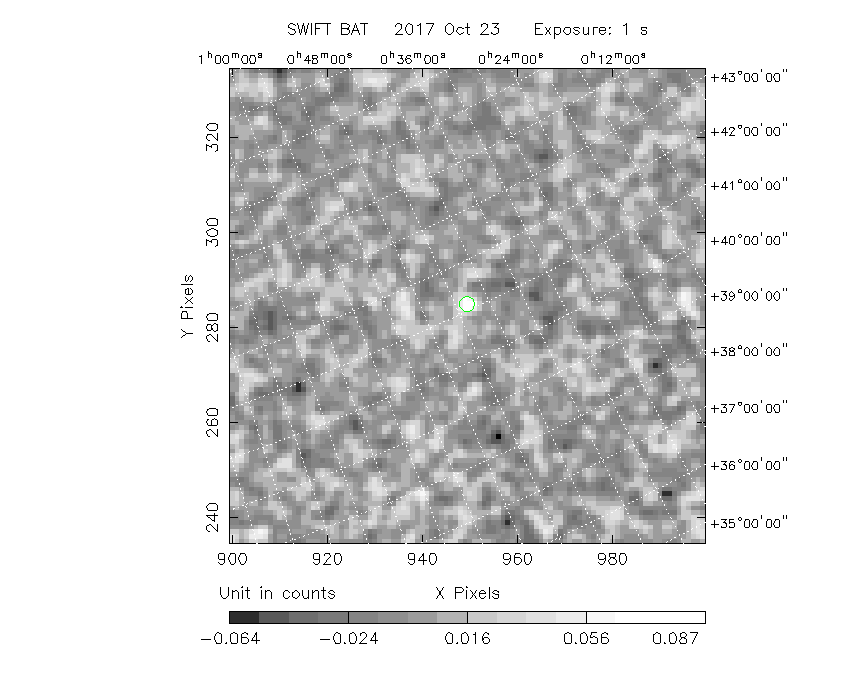```#   RAcent  DECcent POSerr  Theta    Phi     Peak Cts     SNR  Name
10.6593 +41.1435 0.0313   23.2  110.0       0.0997     6.1  TRIG_00781740
------------------------------------------

Foreground time interval of the image:
-0.024   0.996 (delta_t =   1.020 [sec])
Background time interval of the image:
-128.459  -0.024 (delta_t = 128.435 [sec])
```

### Notes:

• 1) All plots contain as much data as has been downloaded to date.
• 2) The mask-weighted light curves are using the flight position.
• 3) Multiple plots of different time binning/intervals are shown to cover all scenarios of short/long GRBs, rate/image triggers, and real/false positives.
• 4) For all the mask-weighted lightcurves, the y-axis units are counts/sec/det where a det is 0.4 x 0.4 = 0.16 sq cm.
• 5) The verticle lines correspond to following: green dotted lines are T50 interval, black dotted lines are T90 interval, blue solid line(s) is a spacecraft slew start time, and orange solid line(s) is a spacecraft slew end time.
• 6) Time of each bin is in the middle of the bin.

#### 1 s binning

##### From T0-310 s to T0+310 s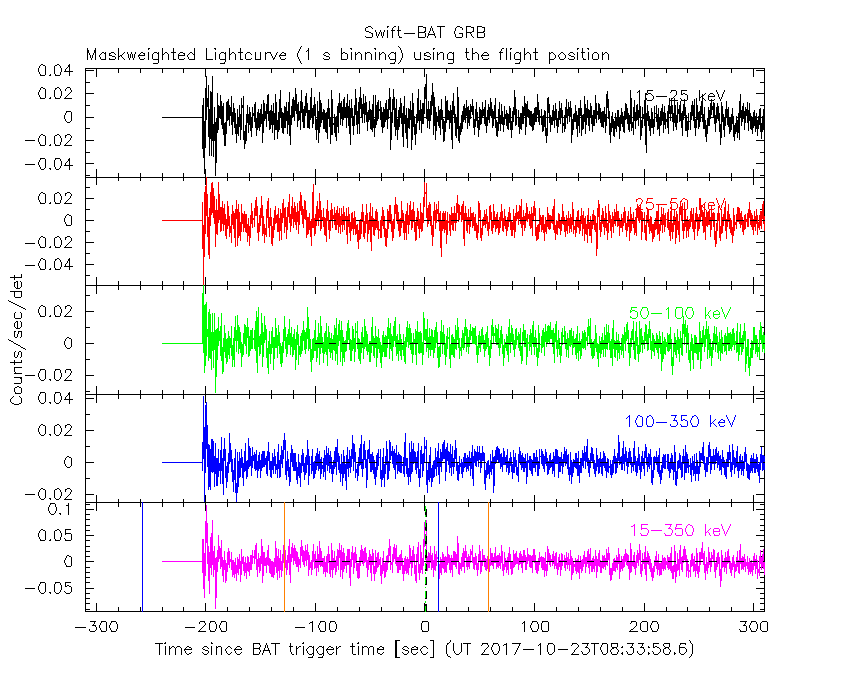##### From T100_start - 20 s to T100_end + 30 s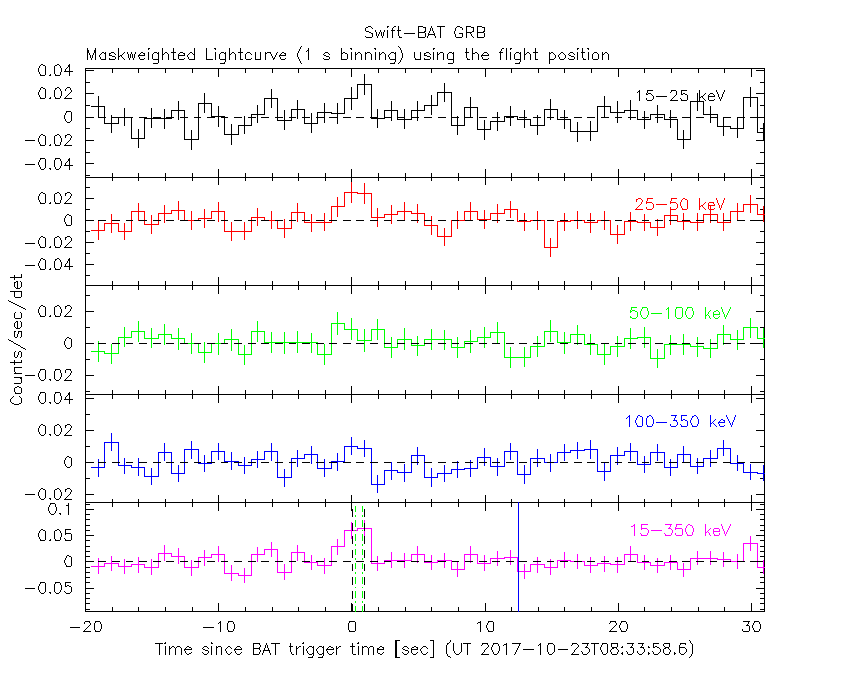##### Full time interval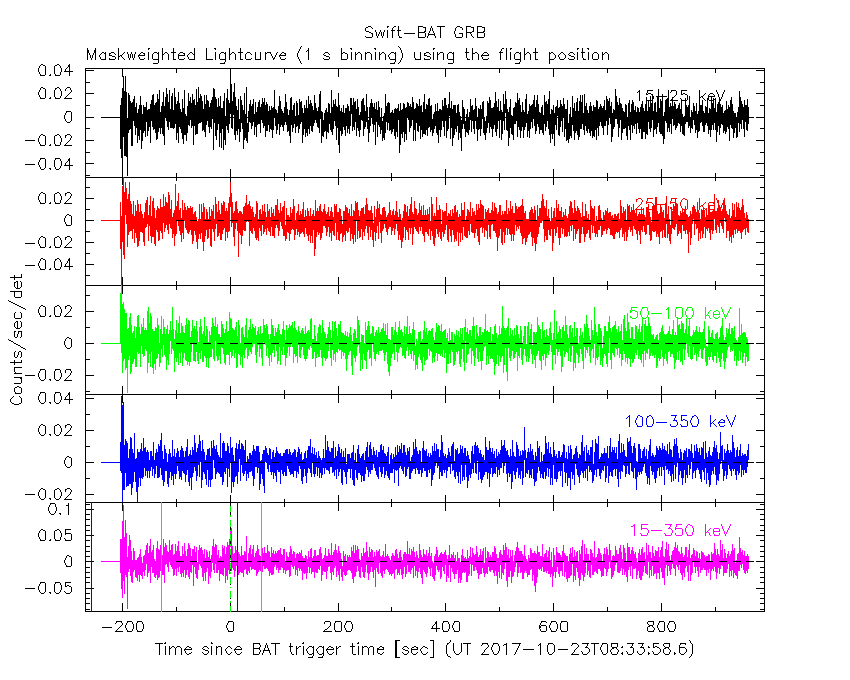#### 64 ms binning

##### Full time interval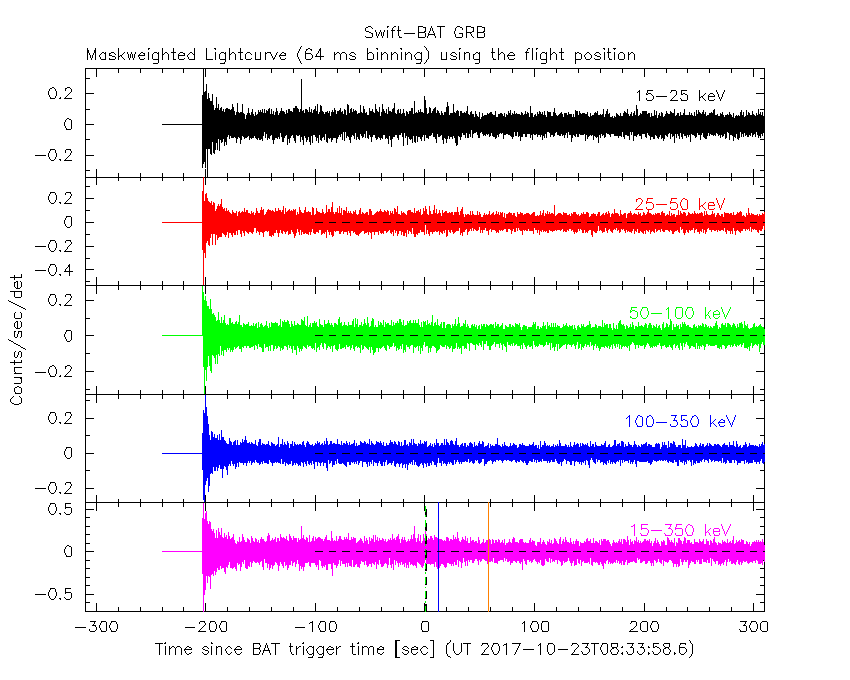##### From T100_start to T100_end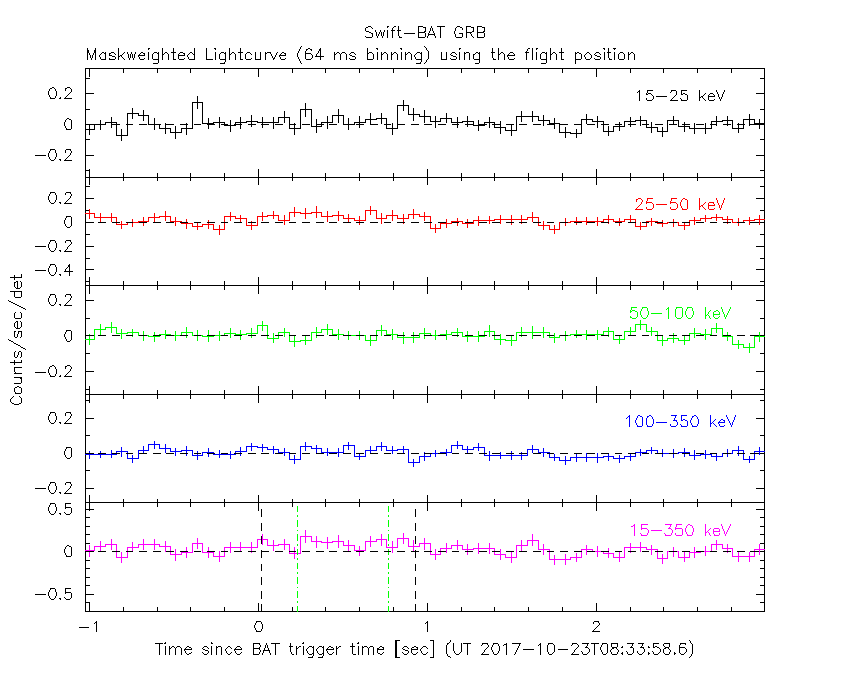##### From T0-5 sec to T0+5 sec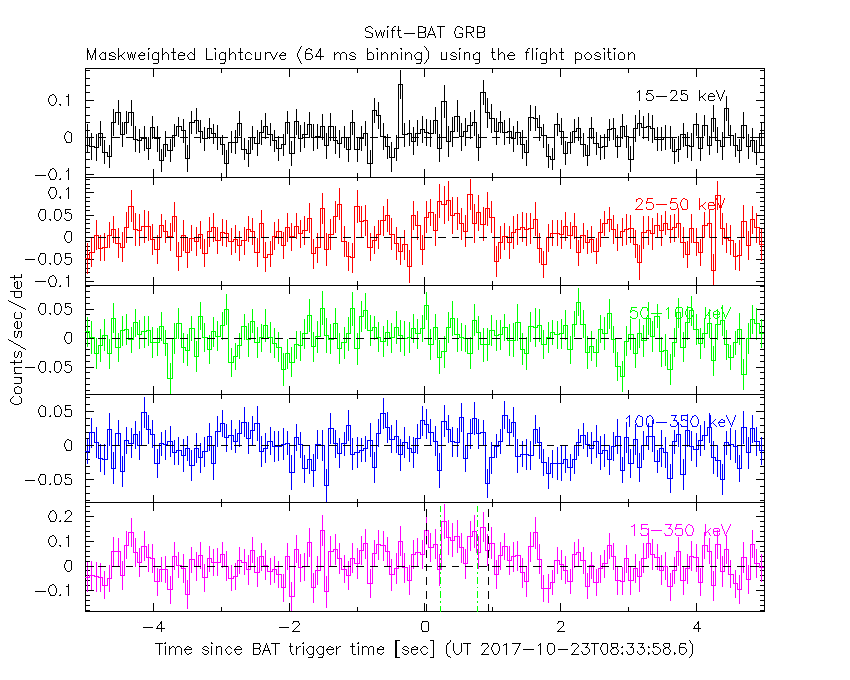#### 16 ms binning

##### From T100_start-5 s to T100_start+5 s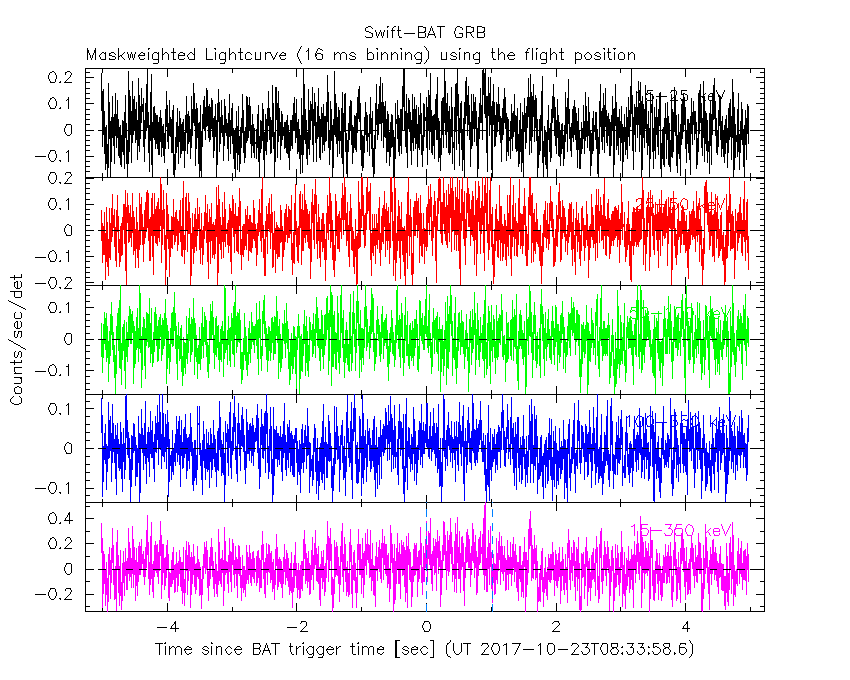#### 8 ms binning

##### From T100_start-3 s to T100_start+3 s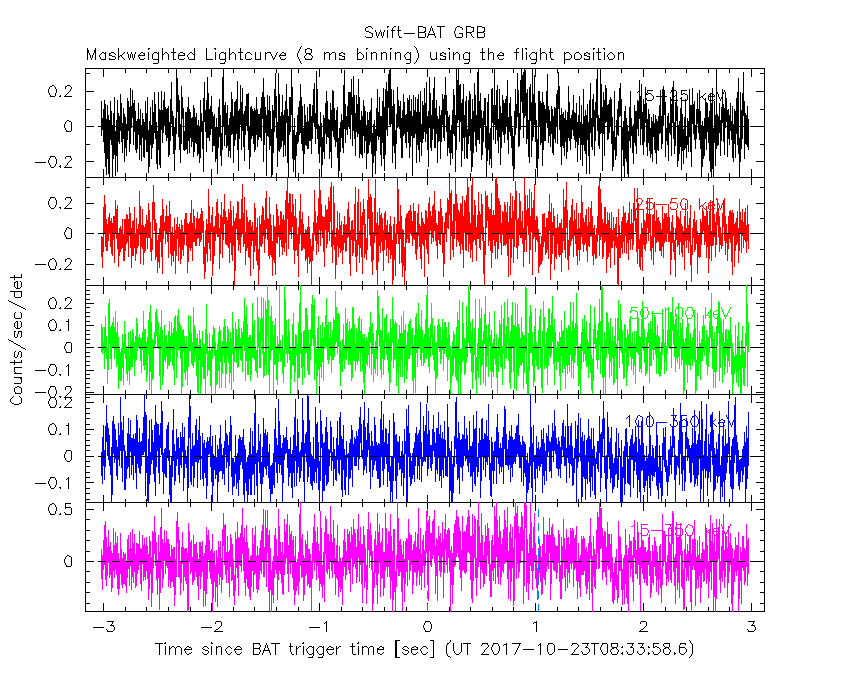#### 2 ms binning

##### From T100_start-1 s to T100_start+1 s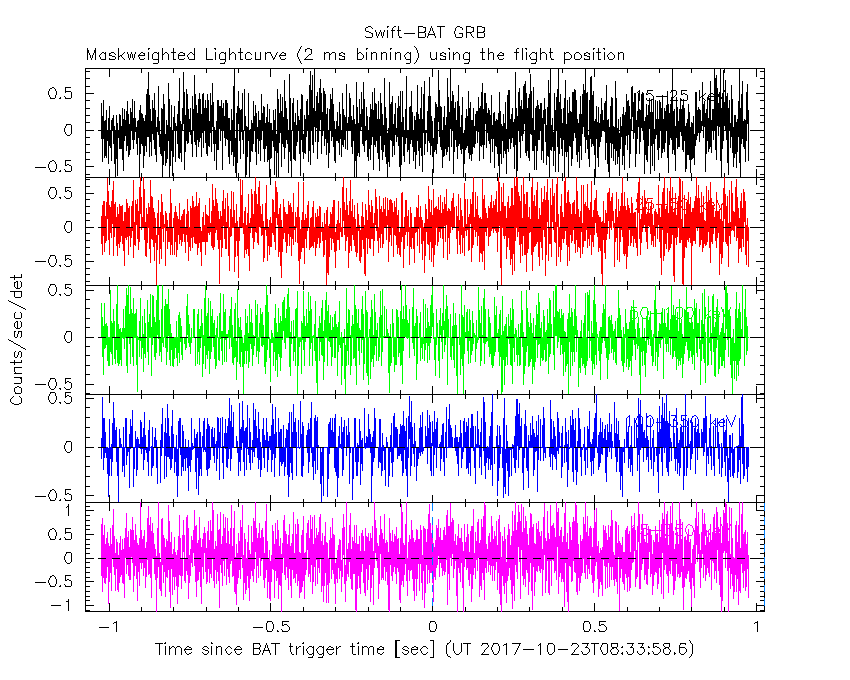#### SN=5 or 10 sec. binning (whichever binning is satisfied first)#### SN=5 or 10 sec. binning (T < 200 sec)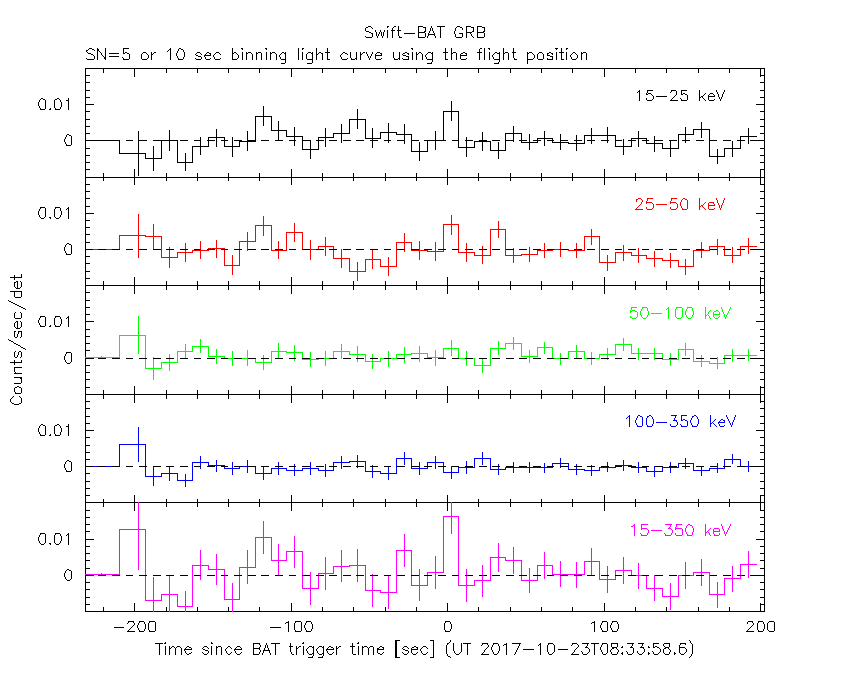### Notes:

• 1) The fitting includes the systematic errors.
• 2) For long bursts, a spectral fit of the pre-slew DRM will introduce some errors in both a spectral shape and a normalization if the PHA file contains a lot of the slew/post-slew time interval.
• 3) For fits to more complicated models (e.g. a power-law over a cutoff power-law), the BAT team has decided to require a chi-square improvement of more than 6 for each extra dof.
• 4) For short bursts (T90<1sec), the specrtum is also fit with Blackbody, OTTB, and Double Blackbody.

#### Time averaged spectrum fit using the pre-slew DRM

##### Power-law model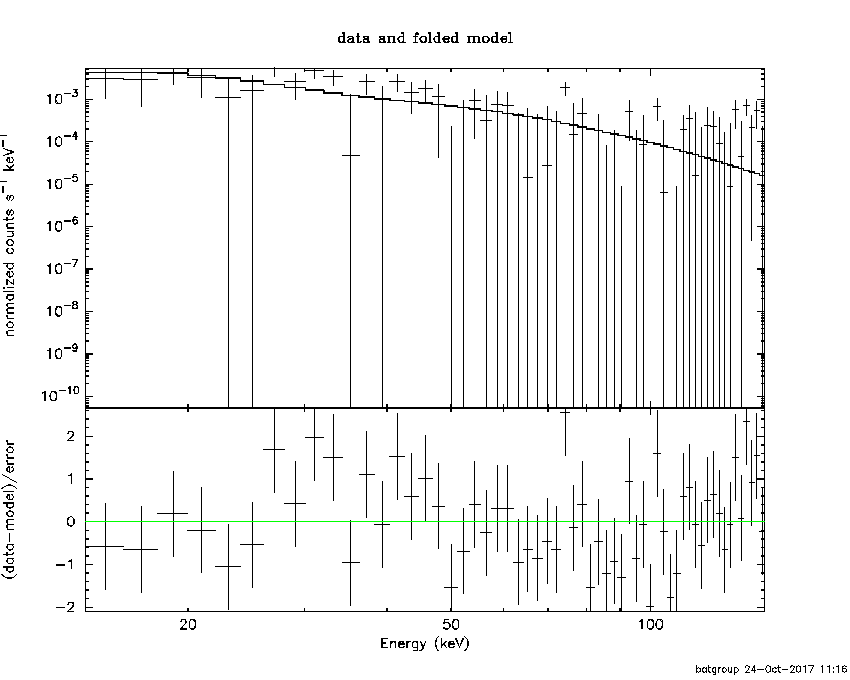```Time interval is from   -0.024 sec. to    0.996 sec.

Spectral model in power-law:
------------------------------------------------------------
Parameters  : value       lower 90%   higher 90%
Photon index: 2.19350     ( -0.436969   0.50628 )
Norm@50keV  : 4.63833E-03    ( -0.00169476   0.00165232 )
------------------------------------------------------------
#Fit statistic : Chi-Squared =          62.95 using 59 PHA bins.
# Reduced chi-squared =          1.104 for     57 degrees of freedom
# Null hypothesis probability =   2.739396e-01

Photon flux (15-150 keV)    in 1.02 sec: 0.765280 ( -0.2094  0.209401 ) ph/cm2/s
Energy fluence (15-150 keV)             : 4.44349e-08 ( -1.40244e-08  1.46805e-08 ) ergs/cm2

```
##### Cutoff power-law model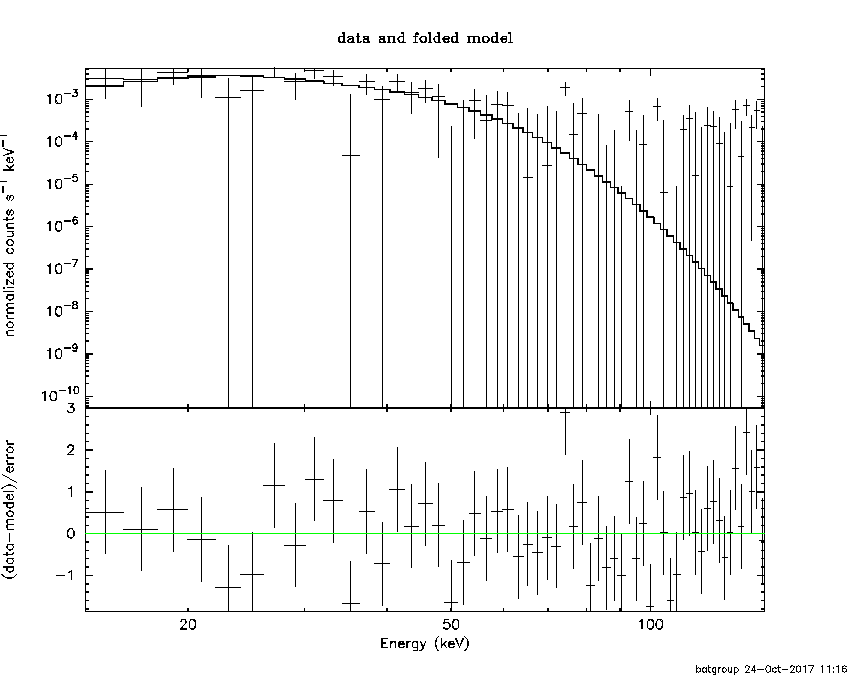```Time interval is from   -0.024 sec. to    0.996 sec.
Spectral model in the cutoff power-law:
------------------------------------------------------------
Parameters  : value       lower 90%   higher 90%
Photon index: -3.29044     ( 2.48868   3.196 )
Epeak [keV] : 34.1918      ( -6.80157 7.15223 )
Norm@50keV  : 13.1253    ( -12.6002   313294 )
------------------------------------------------------------
#Fit statistic : Chi-Squared =          56.49 using 59 PHA bins.
# Reduced chi-squared =          1.009 for     56 degrees of freedom
# Null hypothesis probability =   4.564425e-01

Photon flux (15-150 keV)    in 1.02 sec: 0.743817 ( -0.210046  0.208251 ) ph/cm2/s
Energy fluence (15-150 keV)             : 3.76219e-08 ( -1.04806e-08  1.13868e-08 ) ergs/cm2

```
##### Band function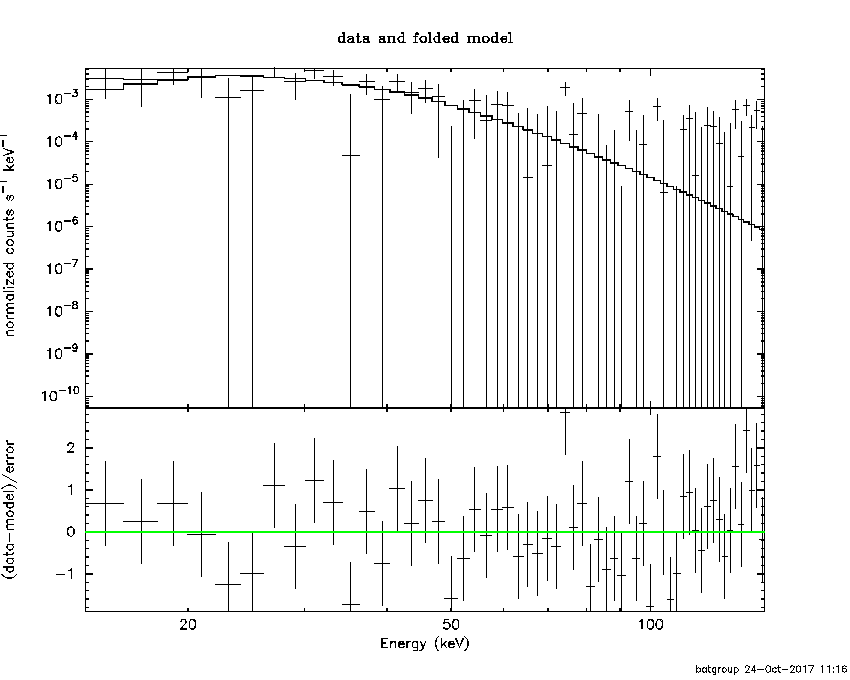```Time interval is from   -0.024 sec. to    0.996 sec.

Spectral model in the Band function:
------------------------------------------------------------
Parameters  : value       lower 90%   higher 90%
alpha       : 4.51390   ( -5.18378   -4.5139 )
beta        : -4.89608    ( 4.89608   2.06645 )
Epeak [keV] : 33.8101      ( -5.39441 5.70462 )
Norm@50keV   : 79.9776    ( -20.1731   602.865 )
------------------------------------------------------------
#Fit statistic : Chi-Squared =          56.56 using 59 PHA bins.
# Reduced chi-squared =          1.028 for     55 degrees of freedom
# Null hypothesis probability =   4.165022e-01

Photon flux (15-150 keV)    in 1.02 sec:  (    ) ph/cm2/s
Energy fluence (15-150 keV)             : 0 ( 0  0 ) ergs/cm2

```
##### Single BB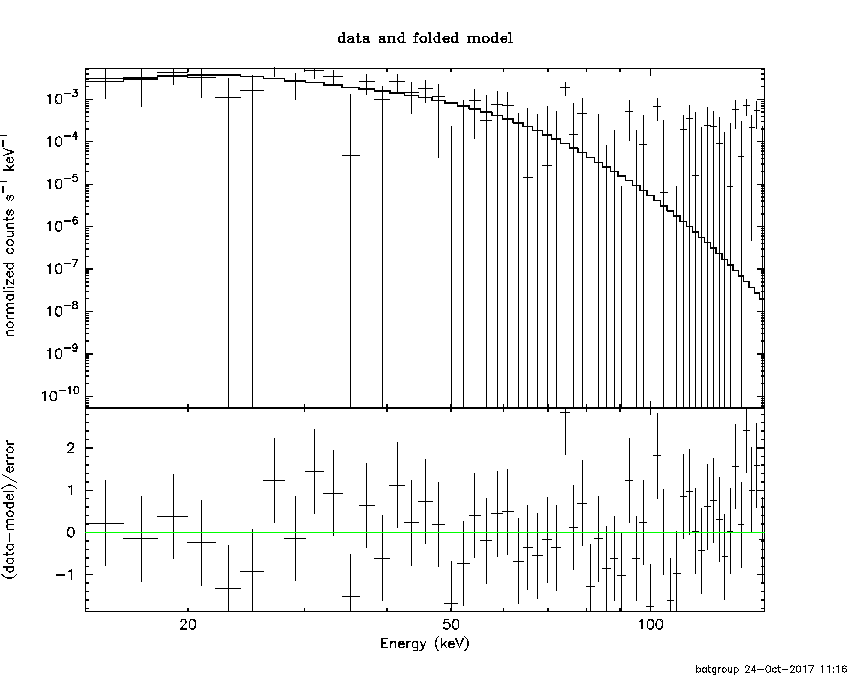```
Spectral model blackbody:
------------------------------------------------------------
Parameters  : value    Lower 90%  Upper 90%
kT [keV]    : 8.74622  (-1.66207  2.01244)
R^2/D10^2   : 0.693454  (-0.401085   0.895457)
(R is the radius in km and D10 is the distance to the source in units of 10 kpc)
------------------------------------------------------------
#Fit statistic : Chi-Squared =          56.77 using 59 PHA bins.
# Reduced chi-squared =         0.9959 for     57 degrees of freedom
# Null hypothesis probability =   4.837441e-01

Energy  Fluence     90% Error
[keV]   [erg/cm2]   [erg/cm2]
15- 25  1.037e-08   4.979e-09
25- 50  2.107e-08   9.551e-09
50-150  7.358e-09   4.735e-09
15-150  3.880e-08   1.777e-08
```
##### Thermal bremsstrahlung (OTTB)```

Spectral model: thermal bremsstrahlung
------------------------------------------------------------
Parameters  : value         Lower 90%    Upper 90%
kT [keV]    : 42.2059       (-17.5407      42.4701)
Norm        : 5.02735       (-1.87075     2.8393)
------------------------------------------------------------
#Fit statistic : Chi-Squared =          60.51 using 59 PHA bins.
# Reduced chi-squared =          1.062 for     57 degrees of freedom
# Null hypothesis probability =   3.502003e-01

Energy  Fluence     90% Error
[keV]   [erg/cm2]   [erg/cm2]
15- 25  1.198e-08   4.952e-09
25- 50  1.612e-08   6.918e-09
50-150  1.322e-08   9.997e-09
15-150  4.132e-08   1.745e-08
```
##### Double BB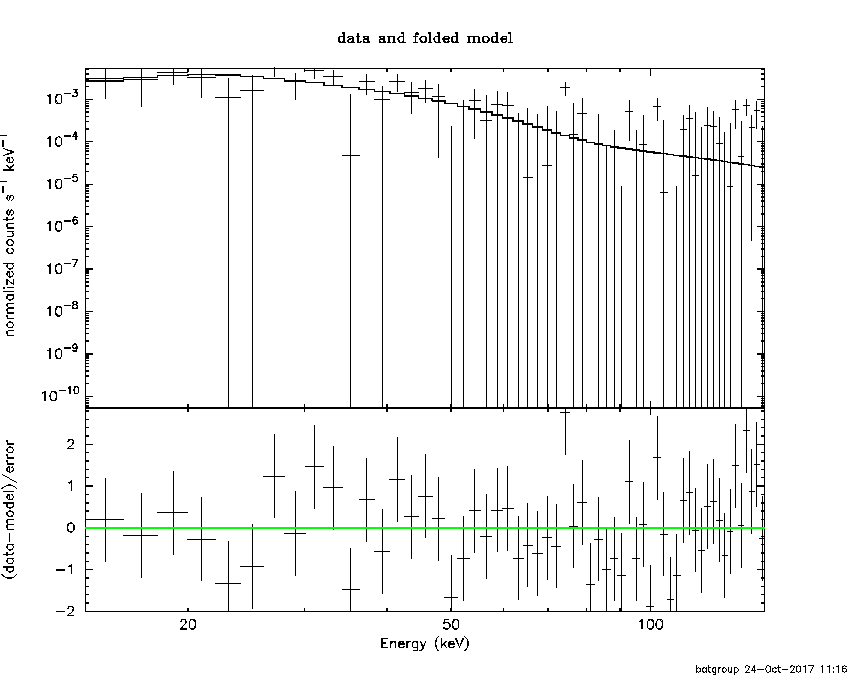```#Fit statistic : Chi-Squared =          56.73 using 59 PHA bins.
#Fit statistic : Chi-Squared =          56.29 using 59 PHA bins.
#Fit statistic : Chi-Squared =          56.27 using 59 PHA bins.
# Reduced chi-squared =          1.032 for     55 degrees of freedom
# Reduced chi-squared =          1.032 for     55 degrees of freedom
# Reduced chi-squared =          1.031 for     55 degrees of freedom
# Reduced chi-squared =          1.023 for     55 degrees of freedom
# Reduced chi-squared =          1.023 for     55 degrees of freedom
# Null hypothesis probability =   4.089468e-01
# Null hypothesis probability =   4.096536e-01
# Null hypothesis probability =   4.104672e-01
# Null hypothesis probability =   4.263768e-01
# Null hypothesis probability =   4.272285e-01

Energy  Fluence     90% Error
[keV]   [erg/cm2]   [erg/cm2]
15- 25  0.000e+00   0.000e+00
25- 50  0.000e+00   0.000e+00
50-150  0.000e+00   0.000e+00
15-150  0.000e+00   0.000e+00
```

#### Peak spectrum fit

##### Power-law model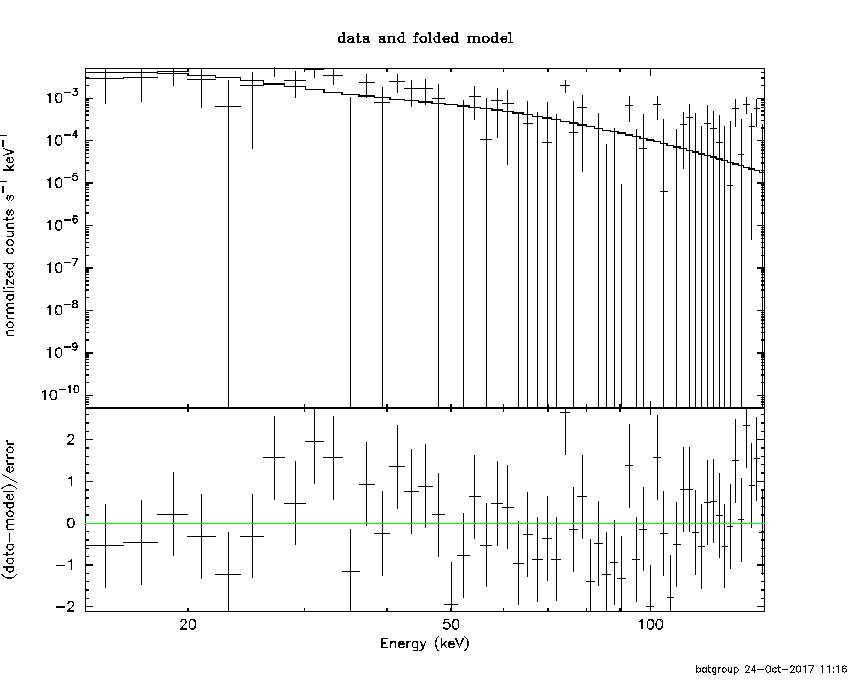```Time interval is from   -0.030 sec. to    0.970 sec.

Spectral model in power-law:
------------------------------------------------------------
Parameters  : value       lower 90%   higher 90%
Photon index: 2.11796     ( -0.457281   0.524301 )
Norm@50keV  : 4.69612E-03    ( -0.00172517   0.00166454 )
------------------------------------------------------------
#Fit statistic : Chi-Squared =          64.63 using 59 PHA bins.
# Reduced chi-squared =          1.134 for     57 degrees of freedom
# Null hypothesis probability =   2.276755e-01

Photon flux (15-150 keV)    in 1 sec: 0.745433 ( -0.21095  0.210984 ) ph/cm2/s
Energy fluence (15-150 keV)             : 4.37158e-08 ( -1.41773e-08  1.47845e-08 ) ergs/cm2

```
##### Cutoff power-law model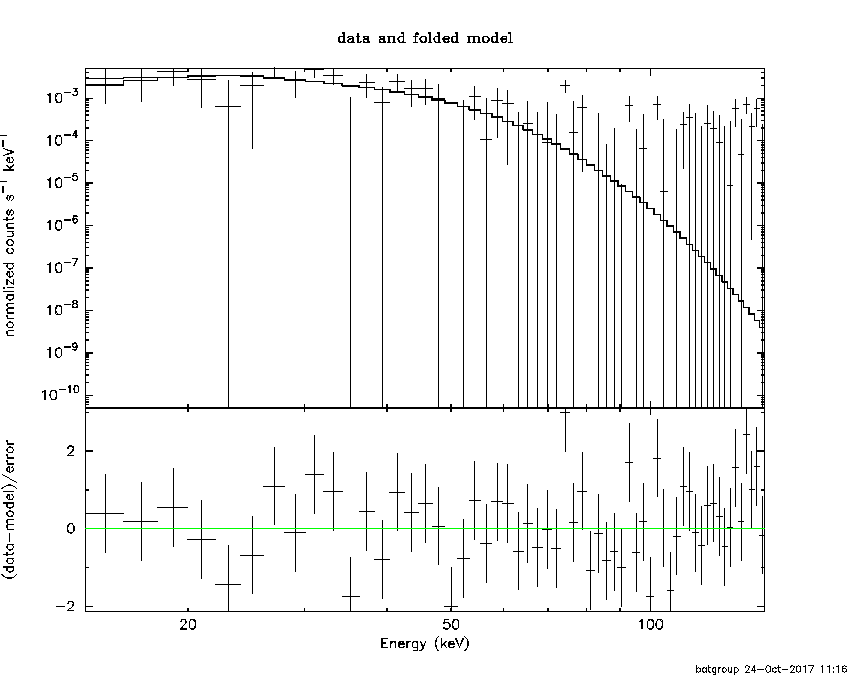```Time interval is from   -0.030 sec. to    0.970 sec.
Spectral model in the cutoff power-law:
------------------------------------------------------------
Parameters  : value       lower 90%   higher 90%
Photon index: -2.84855     ( 1.96169   3.35762 )
Epeak [keV] : 34.4628      ( -6.90025 9.70268 )
Norm@50keV  : 6.35537    ( -5.88218   215026 )
------------------------------------------------------------
#Fit statistic : Chi-Squared =          60.43 using 59 PHA bins.
# Reduced chi-squared =          1.079 for     56 degrees of freedom
# Null hypothesis probability =   3.187987e-01

Photon flux (15-150 keV)    in 1 sec: 0.714728 ( -0.212748  0.21065 ) ph/cm2/s
Energy fluence (15-150 keV)             : 3.56825e-08 ( -1.07253e-08  7.0504e-09 ) ergs/cm2

```
##### Band function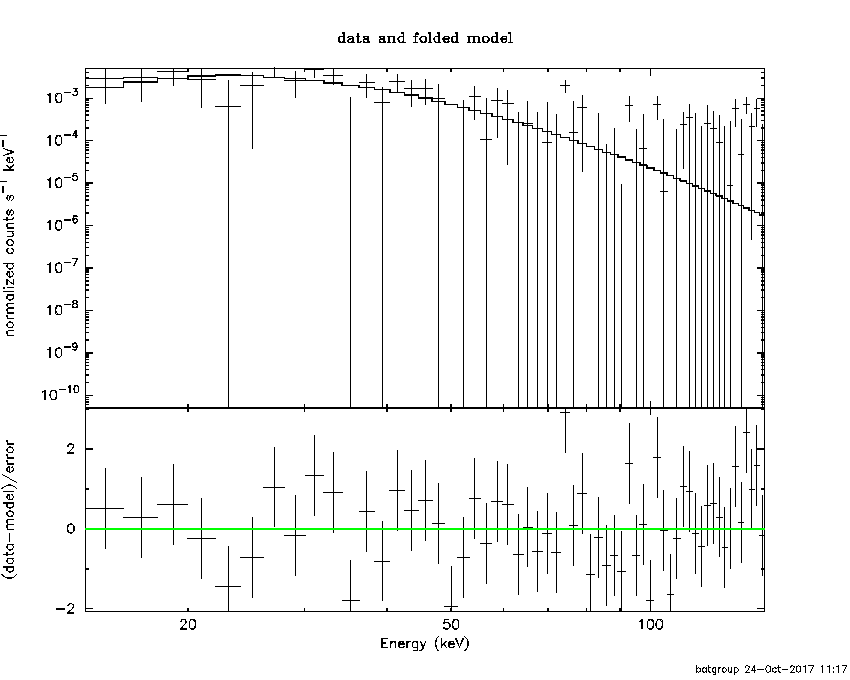```Time interval is from   -0.030 sec. to    0.970 sec.

Spectral model in the Band function:
------------------------------------------------------------
Parameters  : value       lower 90%   higher 90%
alpha       : 3.88369   ( -5.60635   -4.40437 )
beta        : -4.22606    ( 4.25402   1.79967 )
Epeak [keV] : 33.5533      ( -5.83094 7.73277 )
Norm@50keV   : 31.9471    ( -31.8824   54.7787 )
------------------------------------------------------------
#Fit statistic : Chi-Squared =          60.16 using 59 PHA bins.
# Reduced chi-squared =          1.094 for     55 degrees of freedom
# Null hypothesis probability =   2.943188e-01

Photon flux (15-150 keV)    in 1 sec:  (    ) ph/cm2/s
Energy fluence (15-150 keV)             : 3.80684e-08 ( 0  0 ) ergs/cm2

```
##### Single BB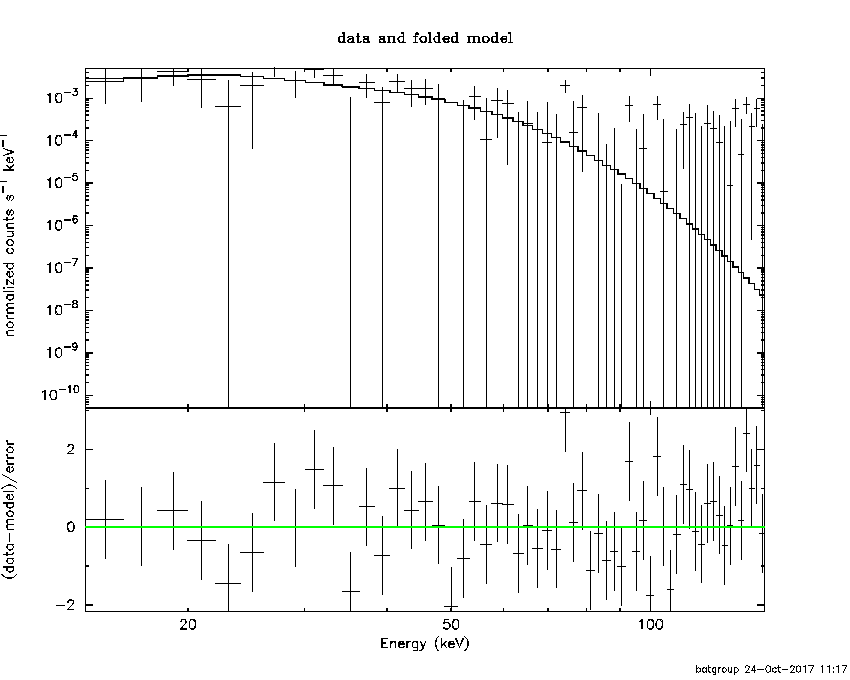```
Spectral model blackbody:
------------------------------------------------------------
Parameters  : value    Lower 90%  Upper 90%
kT [keV]    : 8.87732  (-1.82331  2.28258)
R^2/D10^2   : 0.624416  (-0.382507   0.911353)
(R is the radius in km and D10 is the distance to the source in units of 10 kpc)
------------------------------------------------------------
#Fit statistic : Chi-Squared =          60.53 using 59 PHA bins.
# Reduced chi-squared =          1.062 for     57 degrees of freedom
# Null hypothesis probability =   3.495578e-01

Energy  Fluence     90% Error
[keV]   [erg/cm2]   [erg/cm2]
15- 25  9.511e-09   5.176e-09
25- 50  1.978e-08   1.010e-08
50-150  7.236e-09   5.040e-09
15-150  3.653e-08   2.013e-08
```
##### Thermal bremsstrahlung (OTTB)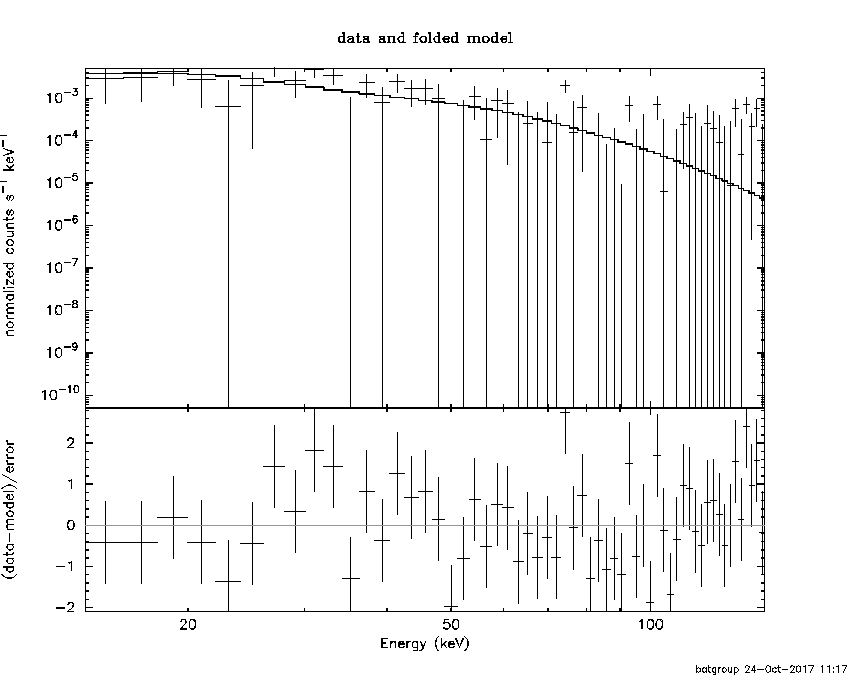```

Spectral model: thermal bremsstrahlung
------------------------------------------------------------
Parameters  : value         Lower 90%    Upper 90%
kT [keV]    : 46.8988       (-21.1822      61.707)
Norm        : 4.55416       (-1.76291     2.74753)
------------------------------------------------------------
#Fit statistic : Chi-Squared =          63.06 using 59 PHA bins.
# Reduced chi-squared =          1.106 for     57 degrees of freedom
# Null hypothesis probability =   2.707745e-01

Energy  Fluence     90% Error
[keV]   [erg/cm2]   [erg/cm2]
15- 25  1.096e-08   4.711e-09
25- 50  1.538e-08   7.526e-09
50-150  1.404e-08   1.017e-08
15-150  4.038e-08   2.086e-08
```
##### Double BB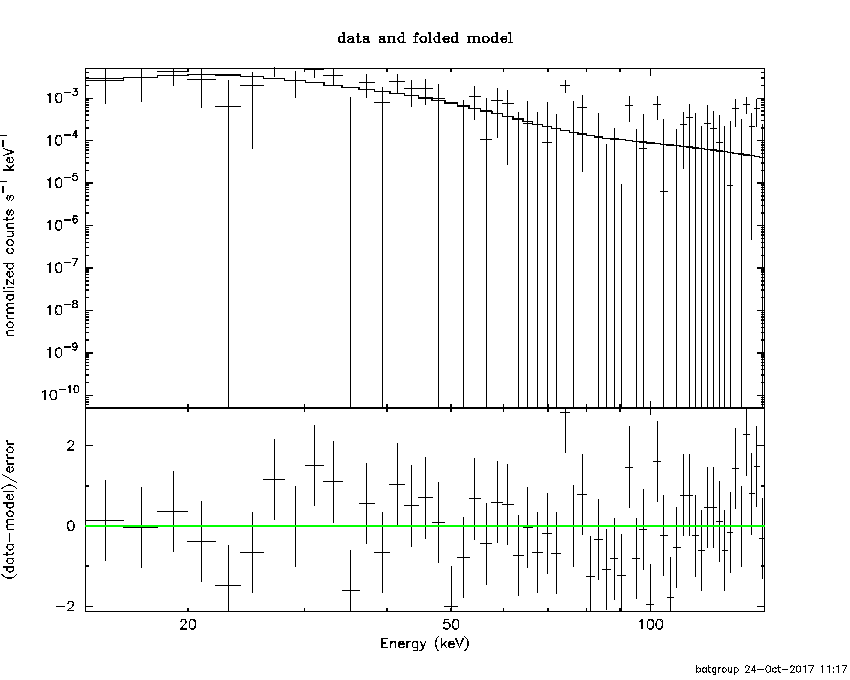```------------------------------------------------------------
#Fit statistic : Chi-Squared =          60.53 using 59 PHA bins.
#Fit statistic : Chi-Squared =          60.50 using 59 PHA bins.
#Fit statistic : Chi-Squared =          59.28 using 59 PHA bins.
#Fit statistic : Chi-Squared =          59.26 using 59 PHA bins.
# Reduced chi-squared =          1.101 for     55 degrees of freedom
# Reduced chi-squared =          1.100 for     55 degrees of freedom
# Reduced chi-squared =          1.078 for     55 degrees of freedom
# Reduced chi-squared =          1.077 for     55 degrees of freedom
# Null hypothesis probability =   2.829916e-01
# Null hypothesis probability =   2.841177e-01
# Null hypothesis probability =   3.221889e-01
# Null hypothesis probability =   3.230234e-01

Energy  Fluence     90% Error
[keV]   [erg/cm2]   [erg/cm2]
15- 25  0.000e+00   0.000e+00
25- 50  0.000e+00   0.000e+00
50-150  0.000e+00   0.000e+00
15-150  0.000e+00   0.000e+00
```
```Plot creation:
Tue Oct 24 11:18:13 EDT 2017
```